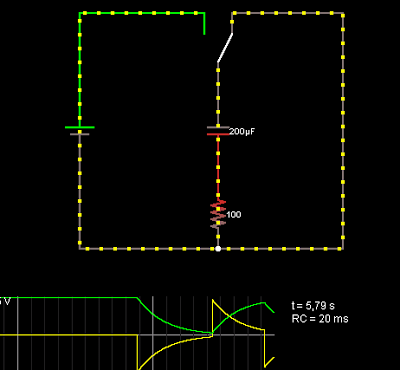## Electronic basics – Capacitor

Basically the capacitor definition is this: “A capacitor (originally known as condenser) is a passive two-terminal electrical component used to store energy in an electric field. The forms of practical capacitors vary widely, but all contain at least two electrical conductors separated by a dielectric (insulator); for example, one common construction consists of metal foils separated by a thin layer of insulating film. Capacitors are widely used as parts of electrical circuits in many common electrical devices.” (wikipedia)
A circuit with a condenser looks like this one:As current flows into the capacitor, the voltage across the capacitor increases. As its voltage approaches the source voltage (the 5V voltage source shown on the left), the current flowing into the capacitor decreases.
After the switch is closed the capacitor will discharge over the resistor and the voltage will look like in the graph.